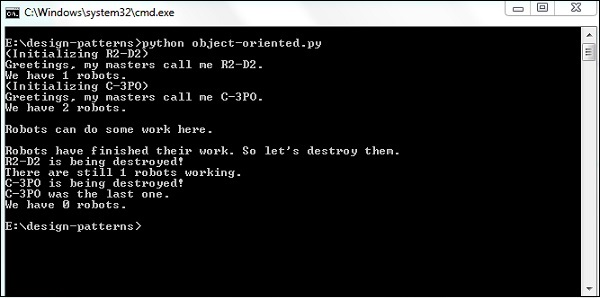# Object Oriented Concepts Implementation

In this chapter, we will focus on patterns using object oriented concepts and its implementation in Python. When we design our programs around blocks of statements, which manipulate the data around functions, it is called procedure-oriented programming. In object-oriented programming, there are two main instances called classes and objects.

## How to implement classes and object variables?

The implementation of classes and object variables are as follows −

```class Robot:
population = 0

def __init__(self, name):
self.name = name
print("(Initializing {})".format(self.name))
Robot.population += 1

def die(self):
print("{} is being destroyed!".format(self.name))
Robot.population -= 1
if Robot.population == 0:
print("{} was the last one.".format(self.name))
else:
print("There are still {:d} robots working.".format(
Robot.population))

def say_hi(self):
print("Greetings, my masters call me {}.".format(self.name))

@classmethod
def how_many(cls):
print("We have {:d} robots.".format(cls.population))
droid1 = Robot("R2-D2")
droid1.say_hi()
Robot.how_many()

droid2 = Robot("C-3PO")
droid2.say_hi()
Robot.how_many()

print("\nRobots can do some work here.\n")

print("Robots have finished their work. So let's destroy them.")
droid1.die()
droid2.die()

Robot.how_many()```

### Output

The above program generates the following output −### Explanation

This illustration helps to demonstrate the nature of class and object variables.

• “population” belongs to the “Robot” class. Hence, it is referred to as a class variable or object.

• Here, we refer to the population class variable as Robot.population and not as self.population.